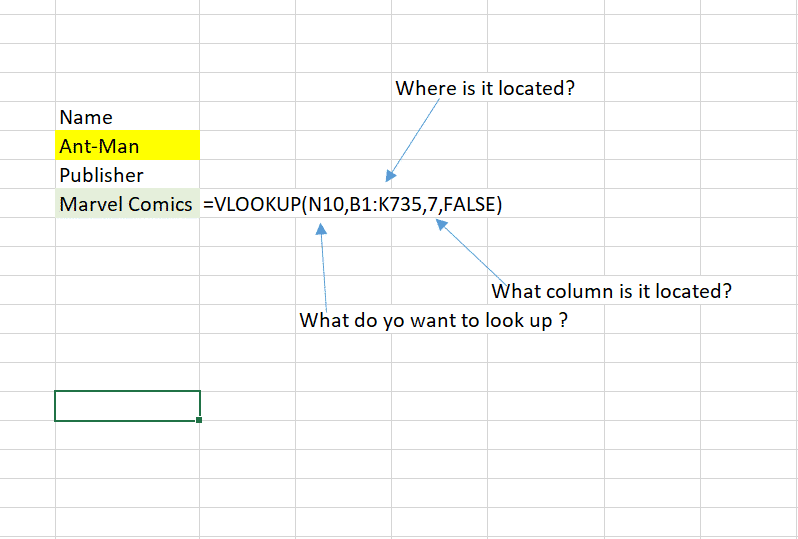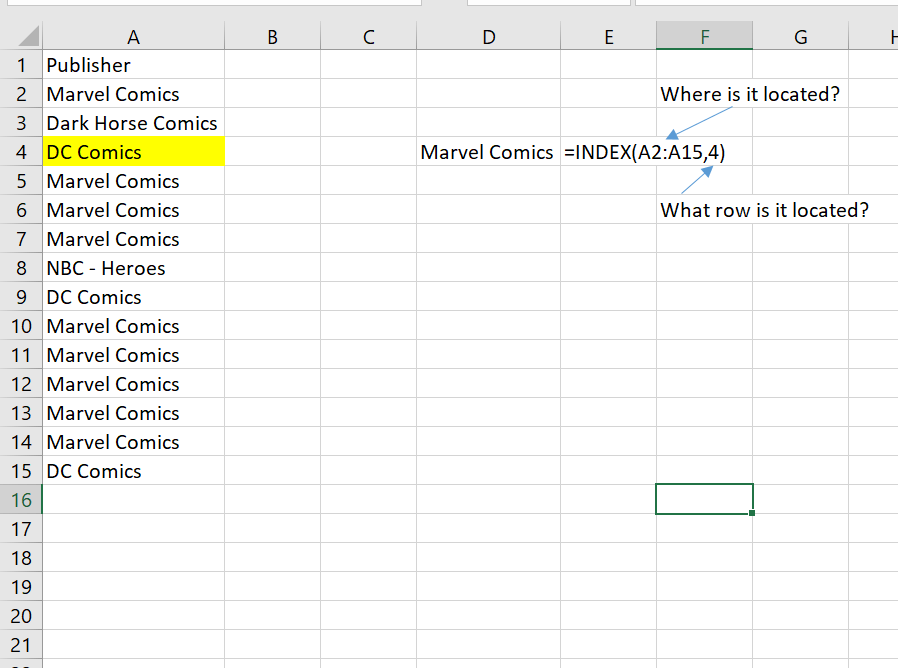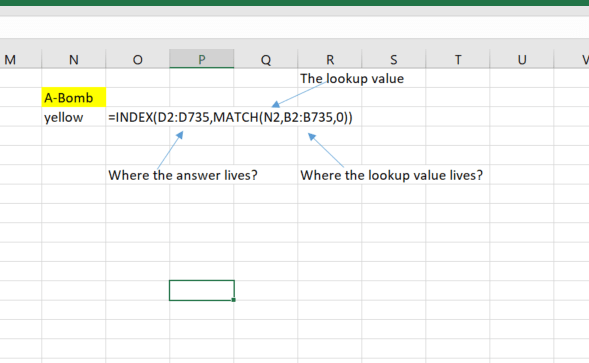# Vlookup vs Index Match

Vlookup is probably one of the top 5 functions that asked for Excel professionals to demonstrate for analysis or while interviewing. I can personally attest that early in my career I was asked whether I could do Vlookup hundreds of times. I often wonder why VLOOKUP became the go-to function for looking up data when Excel has a number of functions such as INDEX MATCH, HLOOKUP and LOOKUP. However, let’s keep our focus on VLOOKUP vs INDEX MATCH since these are the most commonly used lookup functions.

### Why is VLOOKUP used?

Vlookup is used to find corresponding data in an adjacent column.  The formula consists of VLOOKUP (value, table, col_index, [range_lookup])

• a single formula to look up values
• quickly find correlated data
• performs exact and rough matches

• slow
• only provides data in a column
• a single value must be in the row
• data types should be the same in both columns
• cannot lookup values from the left

Let show you how the basic Vlookup formula works. with a superhero data set.

Components of a VLOOKUP:
• value – What you want to lookup? This value has to be in the first column of a table.
• table – Where this information can be found? This the table from which to retrieve a value.
• col_index –  What alise is it on? The column in the table from which to retrieve a value.
• range_lookup – How accurate to do you want it to be? Exact Match or Approximate Match### INDEX MATCH

This is not a single function but a combination of functions. The index function provides a row location of a value in a column or array.  The Match function tells you which row a value is located. So. for example if we want to know what row number a value is located,  it will return it.

### Why is INDEX MATCH used?

Index Match eliminates a lot of the restrictions that Vlookup does. The look value doesn’t have to be in the leftmost table. You can simply use the row number of the value you want and match a value in another column to that row number.

INDEX Function

This function returns a value based on a row number. This is essentially the opposite of the MATCH function.INDEX MATCH

This is a combination of the two functions that allows you to have a better alternative than the VLOOKUP function. The reason this function is better than VLOOKUP is for the following reasons. let us take a look at the formula for  INDEX MATCH which is a combination of both functions:

Components of INDEX MATCH

INDEX (array, MATCH (lookup value, array, 0)) essentially this is INDEX (answer you want to return MATCH (lookup valuewhere that value is located, 0))

• INDEX function —  The function requires the index or row number of the value you are interested in.
• MATCH function: The function gives the index or row number of the value you are interested in.
• value–this is the actual value you want to look
• array-where this value lives.#### Gaelim Holland

Subscribe
Notify of